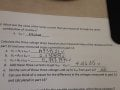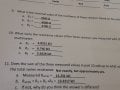# [Lab/Multimeter help] The Sum of The Individual Resistors Not Equal to Measured Series Resistance

#### Creasion

Joined Sep 7, 2019
5
Yea, basically there was an assignment based on the lab that we did.
We measured three values of resistors individually using the multimeter.
Then we measured the Rseries resistance using the multimeter.

On adding up the individual values of the resistors we find out the the value is not exactly the same as the Rseries measurement.

The question then asks " why do you think the answer is different? "

#### MrChips

Joined Oct 2, 2009
29,853
Welcome to AAC!

This is a classic introductory level experiment in electricity, electronics and application of Ohm's Law and circuit theory.

1) To begin, in order to analyze your situation you have to publish your results obtained.

2) Since this is Homework Help, you have to show how you would answer the question. We are here to guide you, not do your homework for you.

#### crutschow

Joined Mar 14, 2008
33,359
What is the measurement accuracy of the meter?

#### WBahn

Joined Mar 31, 2012
29,517
Yea, basically there was an assignment based on the lab that we did.
We measured three values of resistors individually using the multimeter.
Then we measured the Rseries resistance using the multimeter.

On adding up the individual values of the resistors we find out the the value is not exactly the same as the Rseries measurement.

The question then asks " why do you think the answer is different? "

Even if this wasn't homework (which it is), you've given us nothing to go on. What were the original measurement? What what the series measurement? How were the resistors placed in series? How far off were the results from what you expected?

What is your best estimate of why they were different?

Present your findings and your best attempt at an answer and we can serve as a sounding board for you.

#### dl324

Joined Mar 30, 2015
16,162
Also mention the type of meter (digital or analog), the model number, and range(s) being used.

#### Creasion

Joined Sep 7, 2019
5
Ok, sorry for the wait.

Umm, I can't tell you the model or whatever but it's a digital multimeter.

So here's the data:

The arrangement is a simple series connection of resistors:

Color code:

R1: Orange White Red Gold
R2: Blue Grey Red Gold
R3: Red Violet Red Gold

R1: 3.8231 K ohms
R2: 6.746 K ohms
R3: 2.6626 K ohms

Rseries: 13.231 K ohms

5.0005V

R1: 1.447 V
R2: 2.5497 V
R3: 1.0065 V

Current reading - 375.12 micro Amps

Here are the specific questions i'm stumped on ; no 11 c. and 14 f.#### Creasion

Joined Sep 7, 2019
5
Also the Vtot from part 12 refers to the measured multimeter voltage, which is 5.0005 V

#### MrChips

Joined Oct 2, 2009
29,853
Why don't you go over all your numbers and look for any discrepancies.
Perhaps this is an exercise in more than Ohm's Law and circuit analysis.
Perhaps this is an exercise in data collection, measurement, recording, and error analysis?

#### WBahn

Joined Mar 31, 2012
29,517
Ok, sorry for the wait.

Umm, I can't tell you the model or whatever but it's a digital multimeter.

So here's the data:

The arrangement is a simple series connection of resistors:

Color code:

R1: Orange White Red Gold
R2: Blue Grey Red Gold
R3: Red Violet Red Gold

R1: 3.8231 K ohms
R2: 6.746 K ohms
R3: 2.6626 K ohms

Rseries: 13.231 K ohms

5.0005V

R1: 1.447 V
R2: 2.5497 V
R3: 1.0065 V

Current reading - 375.12 micro Amps

Here are the specific questions i'm stumped on ; no 11 c. and 14 f.

View attachment 185733 View attachment 185734
Reading between the lines, it appears that your meter is a 4.5 digit meter (probably a bench meter) that has a max reading on a given scale that starts with a 2 (e.g., it will real 29.999 Ω on the 30 Ω scale but if you go up to 30 Ω it will read 30.00 Ω because it is now on the 300 Ω scale).

Both your R2 and total Rseries measurements are on the 30 kΩ scale and are only reported to the nearest ohm, but your R1 and R3 measurements are on the 3 kΩ scale and are reported to the nearest 0.1 Ω. You can't make ANY assumptions about what the digits would be after the last one shown and, in fact, you can't assume much about the last digit. And that's before you start talking about the accuracy and precision of the meter itself on each range (and these will likely be different from one range to the next).

I doubt you can reasonable expect measurements to be better than 0.1%, especially if you are putting them in series via a breadboard or alligator clips.

As for the voltage readings, what the total voltage measured with the resistors all in place and drawing current, or was it measured open-circuit with no load?

#### WBahn

Joined Mar 31, 2012
29,517
View attachment 185736

No wonder students get confused. Kirchoff would turn in his grave.
Same thing caught my attention. Probably just a typo, but that's not a certainty.

#### crutschow

Joined Mar 14, 2008
33,359
A good thing to remember is that the meter is always part of the load, which will cause an error (usually small and often negligible but must be considered).
So when measuring voltage you need to account for the load current caused by the input impedance of the meter, which will usually slightly reduce the voltage, and when measuring current you need to account for the series impedance it adds, which will usually slightly reduce the current.

#### MrChips

Joined Oct 2, 2009
29,853
The internal resistances of the voltmeter and ammeter has to be taken into account. However, in this case I don't think they come into play as yet.

The first thing to note is that the current reading of 375.12μA does not jive. So there is an error right there.
Hence the measurements, current, voltage, or resistance are in error somewhere.

#### The Electrician

Joined Oct 9, 2007
2,953
Reading between the lines, it appears that your meter is a 4.5 digit meter (probably a bench meter) that has a max reading on a given scale that starts with a 2 (e.g., it will real 29.999 Ω on the 30 Ω scale but if you go up to 30 Ω it will read 30.00 Ω because it is now on the 300 Ω scale).
The R1 reading was 3.8231 K, which would suggest a maximum count of at least 39999. There was a voltage reading of 5.0005 V, which would suggest a maximum count of at least 59999, assuming the meter has the same resolution on voltage as on resistance measurement.

#### The Electrician

Joined Oct 9, 2007
2,953
The TS has a result for V1 = R1 x Itot of 1.434121Apparently the instructor hasn't taught the students about spurious digits, and not to just write down all the digits they see on their calculator.

#### WBahn

Joined Mar 31, 2012
29,517
The R1 reading was 3.8231 K, which would suggest a maximum count of at least 39999. There was a voltage reading of 5.0005 V, which would suggest a maximum count of at least 59999, assuming the meter has the same resolution on voltage as on resistance measurement.
You're right. I've seen meters with 1 and 2 and 9 as the max leading digit. I don't recall seeing max leading digits of 4 or 5, but perhaps this is one. I don't know why the TS can't tell us the make/model of the meter or at least something else about it.

#### WBahn

Joined Mar 31, 2012
29,517
The TS has a result for V1 = R1 x Itot of 1.434121Apparently the instructor hasn't taught the students about spurious digits, and not to just write down all the digits they see on their calculator.
Very good point -- and all too common. I didn't even look at that because I generally figure there's not much point in going past the first or second error until those are rectified since, in doing so, often a lot of other errors disappear as well.

#### MrChips

Joined Oct 2, 2009
29,853
Post #6 lists Multimeter reading - 5.0005V.

Is this the voltage at the power supply with no load connected?
What is the voltage with all three resistors connected?
What is the internal resistance of the power supply?

This is a classic example of the consequences when students do not analyze their results in the lab.
Had the discrepancy been discovered in the lab, the student could have taken the opportunity to investigate the validity of the equation,

V = I x R

on a single resistor. Hopefully then one would have had a better insight on the discrepancy.